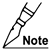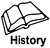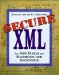# 19.2 Transformation Algorithms

A Transform element appears as the child of the Transform element, which in turn can appear as the child of a Reference, RetrievalMethod, or CipherReference. (See Figure 19-1.) As implicit input, these elements take the output of their previous Transform sibling or, if none exists, the data obtained by dereferencing the URI attribute of their parent.The Transform element, while identical in structure in XML Signature and XML Encryption, is considered, for those recommendations, different and to reside in their respective different namespaces. The Transform element, however, is always found in the XML Signature namespace.

#### 19.2.1 Canonicalization

You can also use any algorithm listed in Section 19.1 as a transform algorithm. The identifier is the same regardless of the role filled by the algorithm.

#### 19.2.2 Base-64 Decoding

` Base64 Identifier:     http://www.w3.org/2000/09/xmldsig#base64 `

The normative specification for base-64 decoding transforms is [RFC 2045]. The base-64 Transform element takes no explicit parameter content; rather, the algorithm decodes the implicit input. This transform is useful if an application needs to access the raw data associated with the encoded content of an element. Its implementation is mandatory.

This transform requires an octet stream for input. If an XPath node-set (or sufficiently functional alternative) is given as input, then it is converted to an octet stream by performing operations logically equivalent to the following steps:

1. Apply an XPath transform with the expression

` self::text() `
2. Take the string value of the node-set.

Thus, if an XML element is identified by a bare name XPointer (see Chapter 7) in the Reference URI, and its content consists of character data encoded in base-64, this transform automatically does the following:

• Strips away the start and end tags of the identified element and any of its descendant elements

• Strips away any descendant comments and processing instructions

The output is an octet stream.

#### 19.2.3 XPath Filtering

` XPath Identifier:     http://www.w3.org/TR/1999/REC-xpath-19991116 `

The normative specification for XPath expression evaluation is [Xpath]. The XPath expression to be evaluated appears as the character content of a transform parameter child element named XPath in the XMLDSIG namespace. Implementation of this transform is recommended. The DTD for the XPath element follows:

` <!-- XPath DTD --> <!ELEMENT XPath (#PCDATA) > `

In schema notation, it has the following form:

` <!-- XPath Schema --> <element name="XPath" type="string"/> `
##### XPath Transform Input

The input required by this transform is an XPath node-set. If the actual input is an XPath node-set resulting from a null URI or bare name XPointer dereference, then comment nodes will have been omitted. If the actual input is an octet stream, then the application must convert the octet stream to an XPath node-set suitable for use by Canonical XML with comments. (A subsequent application of the required Canonical XML algorithm would strip away these comments.) In other words, the input node-set should be equivalent to the one created by the following process:

1. Initialize an XPath evaluation context by setting the initial node equal to the input XML document's root node, and set the context position and size to 1.

2. Evaluate the following XPath expression:

` (//. | //@* | //namespace::*) `

The evaluation of this expression includes all of the document's nodes (including comments) in the node-set.

##### XPath Transform Output

The transform output is also an XPath node-set. The XPath expression appearing in the XPath parameter is evaluated once for each node in the input node-set. The result is converted to a Boolean value. If the Boolean value is true, then the node is included in the output node-set. If the Boolean value is false, then the node is omitted from the output node-set. In effect, the XPath expression is an XPath predicate except that the usual surrounding square brackets are omitted.

This transform ensures that only specifically defined changes to the input XML document are permitted after the signature is affixed. To do so, omit those nodes that are allowed to change, and include all other input nodes in the output. The XPath expression author is responsible for including all nodes whose changes should affect the validity of signatures in their application context.

An example would be a document requiring two enveloped signatures. Each signature must omit itself from its own digest calculations. It is also necessary to exclude the second signature element from the digest calculations of the first signature to ensure that adding the second signature does not break the first signature. Thus the EnvelopedSignature transform (see Section 19.2.4) is inadequate for the second signature, but probably adequate for the first signature.

##### XPath Transform Evaluation

The XPath transform establishes the following evaluation context for each node of the input node-set:

• A context node equal to a node of the input node-set.

• A context position, initialized to 1.

• A context size, initialized to 1.

• A library of functions equal to the function set that XPath defines plus a function named "here( )" (see the XPath Function "here()" later in this chapter).

• A set of variable bindings. No means for initializing these bindings is defined, so the set of variable bindings used when evaluating the XPath expression is empty. Use of a variable reference in the XPath expression, therefore, results in an error.

• The set of namespace declarations in scope for the XPath expression.

As a result of the context node setting, the XPath expressions appearing in this transform will be quite similar to those used by XSLT. The only difference is that the size and position are always 1, reflecting the fact that the transform automatically visits every node. (In XSLT, you recursively call the command "apply-templates" to visit the nodes of the input tree.)

Even if comments were removed from the input node-set, the comment nodes still exist in the underlying parse tree and can separate text nodes. For example, the markup

` <e>Hello, <!-- comment --> world!</e> `

contains two text nodes. Therefore, the expression

` self::text()[string()="Hello, world!"] `

would fail. Should this problem arise in an application, you can solve it in one of two ways:

• By converting the document to Canonical XML before the XPath transform, to physically remove the comments and concatenate resulting adjacent text

• By matching the node based on the parent element's string value

For example, using the expression

` self::text()[string(parent::e)="Hello, world!"] `

would work.

##### XPath Function "here( )"

The function "here( )" is defined as follows:

` node-set here( ) `

The "here( )" function returns a node-set containing the attribute or processing instruction node or the parent element of the text node that directly bears the XPath expression. This expression results in an error if the containing XPath expression does not appear in the same XML document against which the XPath expression is being evaluated. It works in the same way as the "here( )" function added by XPointer (see Chapter 7).

##### XPath Transform Example

As an example, consider the creation of an enveloped signature (a Signature element that is a descendant of an element being signed). Although the signed content should not change after signing, the elements within the Signature element do change (e.g., the digest value must be put inside the DigestValue and the SignatureValue must be subsequently calculated). One way to prevent these changes from invalidating the digest value in DigestValue is to add an XPath transform that omits all Signature elements and their descendants. For example:

` <Document>   ...   <Signature xmlns="http://www.w3.org/2000/09/xmldsig#">     <SignedInfo>       ...       <Reference URI="">         <Transforms>           <Transform Algorithm="http://www.w3.org/TR/1999/REC-xpath-19991116">             <XPath               xmlns:dsig="http://www.w3.org/2000/09/xmldsig#">               not(ancestor-or-self::dsig:Signature)             </XPath>           </Transform>         </Transforms>         <DigestMethod           Algorithm="http://www.w3.org/2000/09/xmldsig#sha1"/>         <DigestValue>...</DigestValue>       </Reference>     </SignedInfo>     <SignatureValue>...</SignatureValue>   </Signature>   ... </Document> `

Due to the null Reference URI in this example, the XPath transform input node-set contains all nodes in the entire parse tree, starting at the root node, except the comment nodes. Each node in this node-set is included in the output node-set except if the node or one of its ancestors has a tag of "Signature" in the XMLDSIG namespace.

A more elegant solution uses the "here( )" function to omit only the Signature containing the XPath transform, thereby allowing enveloped signatures to sign other signatures. In the preceding example, you would use the following XPath element:

` <XPath xmlns:dsig="http://www.w3.org/2000/09/xmldsig#">     count(ancestor-or-self::dsig:Signature |            here()/ancestor::dsig:Signature) >     count(ancestor-or-self::dsig:Signature) </XPath> `

Because the XPath equality operator converts node-sets to string values before comparison, we must instead use the XPath union operator ("|").

For each node of the document, the predicate expression is true if and only if the node-set containing the node and its Signature element ancestors does not include the enveloped Signature element containing the XPath expression. That is, the union must not produce a larger set if the enveloped Signature element is in the node-set given by ancestor-or-self::Signature.

#### 19.2.4 Enveloped Signature Transform

` Enveloped Signature Identifier:     http://www.w3.org/2000/09/xmldsig#enveloped-signature `

An enveloped signature transform removes the entire Signature element containing the transform from the data being transformed. If it appears in a Reference, then that Signature element would generally not be included in the digest calculated. In such a case, the digest calculation removes the entire string of characters that an XML processor uses to match the subdocument of which the Signature element is the apex. The output of the transform is equivalent to the output that would result from replacing this transform with an XPath transform containing the following XPath parameter element:

` <XPath xmlns:dsig="http://www.w3.org/2000/09/xmldsig">     count(ancestor-or-self::dsig:Signature |         here()/ancestor::dsig:Signature) >     count(ancestor-or-self::dsig:Signature) </XPath> `

The input and output requirements of this transform are identical to those of the XPath transform. You do not have to use an XPath expression evaluator to create this transform. However, this transform must produce the same output as the XPath transform parameterized by the XPath expression above. See also Section 19.2.3 on XPath filtering.

#### 19.2.5 XSLT Transform

` XSLT Identifier:     http://www.w3.org/TR/1999/REC-xslt-19991116 `

The normative specification for the XSL transform is [XSLT]. The XSL transformation is encoded within an XSLT namespace-qualified stylesheet element that must be the sole child of the Transform element. Implementation of this transform is optional. The XSLT processing model determines whether it causes in-line processing of XSLT declarations embedded in the input. Applying multiple stylesheets successively may require multiple Transform elements. You can invoke a remote stylesheet by using the XSLT "include" or "import" feature within the stylesheet child of the Transform element.

The DTD for the stylesheet element follows:

` <!-- stylesheet DTD --> <!ELEMENT stylesheet (#PCDATA) > `

In schema notation, it has the following form:

` <!-- stylesheet Schema --> <element name="stylesheet" type="string"/> `

This transform requires an octet stream as input. If the actual input is an XPath node-set, then the signature application should attempt to convert it to octets (i.e., apply Canonical XML), as described in the Transform pipeline model detailed in Chapter 10.

The output of this transform is an octet stream. The [XSLT] specification states the processing rules for the XSL stylesheet or Transform element. We recommend that XSLT transform authors use an output method of "xml" for XML and HTML. XSLT implementations do not produce consistent serializations of their output; for signature purposes, then, you must usually insert a transform after the XSLT transform to canonicalize the output. These steps will help to ensure interoperability of the resulting signatures among applications that support the XSLT transform. Note that if the output is actually HTML, then the result of these steps is logically equivalent to [XHTML].

#### 19.2.6 XPointer

` XPointer Identifier:     http://www.w3.org/2001/04/xmldsig-more/xptr `

This transform algorithm takes an [Xpointer] as an explicit parameter. It provides a more powerful way to extract parts of an XML document to sign, including fail-over provisions. Full implementation of XPointer is optional, however. An example of its use follows, where failure to find a node with an ID of "foo" would fail over to the second search, that for an element named "Zab" in the "urn:baz" namespace with an attribute named "Tag" having the value "foo".

` <Transform   Algorithm="http://www.w3.org/2001/04/xmldsig-more/xptr">   <Xpointer    xmlns="http://www.w3.org/2001/04/xmldsig-more/xptr">     xpointer(id("foo"))     xmlns(bar=urn:baz)     xpointer(//bar:Zab[@Tag="foo"])   </XPointer> </Transform> `

The DTD for the XPointer element follows:

` <!-- XPointer DTD --> <!ELEMENT XPointer (#PCDATA)> `

In schema notation, it has the following form:

` <!-- XPointer Schema --> <element name="XPointer" type="string"/> `

Input to this transform consists of an octet stream (which is then parsed into XML). Output from this transform is a node-set; the results of the XPointer are processed as defined in Chapter 6 for a same-document XPointer.

#### 19.2.7 XML Schema Validation

` Schema Validation Identifier:     http://www.w3.org/TR/2001/09/Validate# `The XML schema validation transform was temporarily included in an intermediate draft of XMLDSIG but currently has its own separate informal document.

This transform provides control over schema validation [Schema]. If no explicit parameters are provided, it indicates that the schema validation must be applied to the implicit input data according to the schema information within that data. If the Transform element has as its sole child a namespace-qualified "schema" element, that element contains the schema to use for validation. The [Schema] processing model determines whether it causes in-line processing of local schema declarations in the input. Applying multiple schemas successively may require multiple Transform elements. You can invoke a remote schema by using the [Schema] "include" or "import" features within the schema child element of the Transform element.

While most of the changes made by [Schema] are additions that are outside the XPath data model, schema validation can affect default attribute values and element content. When this possibility can occur, both the signer and the verifier must do the same schema validation for a signature to be meaningful.

#### 19.2.8 Decryption Transform

Refer back to Chapter 16 for information on the decryption transform.Secure XML: The New Syntax for Signatures and Encryption
ISBN: 0201756056
EAN: 2147483647
Year: 2005
Pages: 186